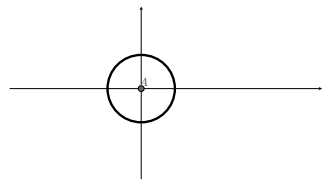# Geogebra to Tikz Scaling

Petrucho shared this question 4 years ago

I try to export simple circle from geogebra to latex with Tikz/pgf export, but it scales very bad and i cant hide numbers on axis even if i toogle off "Show numbers" in options.

Thats the code

\documentclass[10pt]{article}

\usepackage{pgf,tikz,pgfplots}

\pgfplotsset{compat=1.15}

\usepackage{mathrsfs}

\usetikzlibrary{arrows}

\pagestyle{empty}

\begin{document}

\definecolor{rvwvcq}{rgb}{0.08235294117647059,0.396078431372549,0.7529411764705882}

\begin{tikzpicture}[line cap=round,line join=round,>=triangle 45,x=1cm,y=1cm]

\begin{axis}[

x=1cm,y=1cm,

axis lines=middle,

xmin=-2.192771351464916,

xmax=3.900302354670434,

ymin=-1.7555886587865601,

ymax=1.7126141947057099,

xtick={-2,-1.5,...,3.5},

ytick={-1.5,-1,...,1.5},]

\clip(-2.192771351464916,-1.7555886587865601) rectangle (3.900302354670434,1.7126141947057099);

\draw [line width=2pt] (0,0) circle (1cm);

\draw (0.07895422582257174,1.16444839415374) node[anchor=north west] {\textbf{1}};

\draw (-1.2229395504883551,0.20515824318779308) node[anchor=north west] {-1};

\draw (1.17528582692651,0.2631373182461745) node[anchor=north west] {1};

\draw (0.242349800987101,-1.0914647081178275) node[anchor=north west] {-1};

\begin{scriptsize}

\draw [fill=rvwvcq] (0,0) circle (2.5pt);

\draw (0.04205845078541996,0.11291880559491356) node {$A$};

\end{scriptsize}

\end{axis}

\end{tikzpicture}

\end{document}

Files: 2.PNG11

Every picture scales bad. For example simple circle. And second problem is how can i hide numbers on axis in tikz file? If I turn off them in geogebra but they appears in tikz1

Just exporting of the tikz code wont do it. I always have to fiddle with the code afterward.Jour minimal example works fine.

\documentclass[10pt]{article}
\usepackage{pgf,tikz,pgfplots}
\pgfplotsset{compat=1.15}
\usepackage{mathrsfs}
\usetikzlibrary{arrows}
\pagestyle{empty}
\begin{document}
\definecolor{wrwrwr}{rgb}{0.3803921568627451,0.3803921568627451,0.3803921568627451}
\begin{tikzpicture}[line cap=round,line join=round,>=triangle 45,x=1.0cm,y=1.0cm]
\begin{axis}[
x=1.0cm,y=1.0cm,
axis lines=middle,
%ymajorgrids=true,
%xmajorgrids=true,
xmin=-3.899770142167611,
xmax=5.372577304997765,
ymin=-2.6790372195915855,
ymax=2.4374608086866005,
ticks=none,
%xtick={-3.0,-2.0,...,5.0},
%ytick={-2.0,-1.0,...,2.0},
]
\clip(-3.899770142167611,-2.6790372195915855) rectangle (5.372577304997765,2.4374608086866005);
\draw [line width=2.pt] (0.,0.) circle (1.cm);
\begin{scriptsize}
\draw [fill=wrwrwr] (0.,0.) circle (2.5pt);
\draw[color=wrwrwr] (0.06812628792568098,0.19768769222604762) node {$A$};
\end{scriptsize}
\end{axis}
\end{tikzpicture}
\end{document}